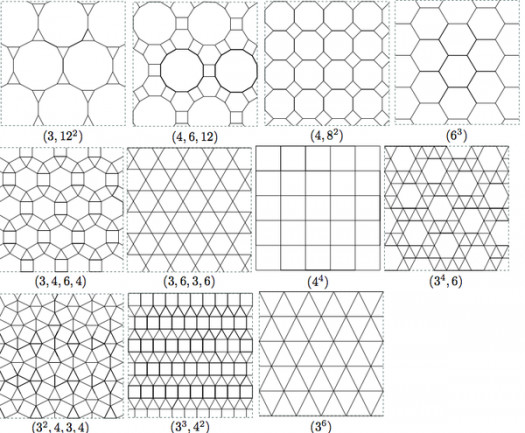# What Do You Know About Lattices?

10 Questions | Total Attempts: 43SettingsA lattice is an abstract structure studied in the mathematical subdisciplines of order theory and abstract algebra. It consists of a partially ordered set in which every two elements have a unique supremum (also called a least upper bound or join) and a unique infimum (also called a greatest lower bound or meet).

• 1.
Lattice is studied in ....?
• A.

Mathematics

• B.

English Language

• C.

History

• D.

Philosophy

• 2.
Lattice consists of how many partially ordered set elements ?
• A.

2

• B.

3

• C.

4

• D.

1

• 3.
The ......... is the only defining identity that is peculiar to lattice theory?
• A.

Absorption law

• B.

Law of physics

• C.

Newton law

• D.

Laws of power

• 4.
The algebraic interpretation of lattices plays an essential role in ......?
• A.

Vector space

• B.

Polytopes

• C.

Linear algebra

• D.

Universal algebra

• 5.
What is the smallest crystal lattice?
• A.

Unit cell

• B.

Primitive cell

• C.

Lattice points

• D.

Basis

• 6.
In a bounded lattice the empty join and the empty meet can also be defined as ....?
• A.

1 and 1

• B.

0 and 1

• C.

2 and 1

• D.

0 and 0

• 7.
Lattice has how many definitions?
• A.

Two

• B.

Three

• C.

Four

• D.

Five

• 8.
Continous lattice is a complete lattice that is continuous as a .....?
• A.

Supremum

• B.

Infimum

• C.

Poset

• D.

Monoid

• 9.
A/an ........ is a complete lattice that is algebraic as a poset?
• A.

Heyting algebra

• B.

Boolean algebra

• C.

Algebraic lattice

• D.

Linear lattice

• 10.
In general, some elements of a ........ might not have a complement, and others might have more than one complement?
• A.

Bounded lattice

• B.

Algebraic lattice

• C.

Continuous lattice

• D.

Complete lattice

Related TopicsBack to top